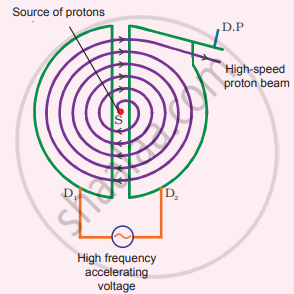Tamil Nadu Board of Secondary EducationHSC Science Class 12th

# Discuss the working of cyclotron in detail. - Physics

Numerical

Discuss the working of cyclotron in detail.

#### Solution

A cyclotron is a device used to accelerate the charged particles to gain large kinetic energy. It is also called a high-energy accelerator. It was invented by Lawrence and Livingston in 1934.

Principle:

When a charged particle moves normal to the magnetic field, it experiences magnetic Lorentz force.

Construction:

The particles are allowed to move in between two semicircular metal containers called Dees (hollow D – shaped objects). Dees are enclosed in an evacuated chamber and it is kept in a region with a uniform magnetic field controlled by an electromagnet. The direction of a magnetic field is normal to the plane of the Dees. The two Dees are kept separated with a gap and the source S (which ejects the particle to be accelerated) is placed at the center in the gap between the Dees. Dees are connected to high frequency alternating potential difference.Working of cyclotron

Working:

Let us assume that the ion ejected from source S is positively charged. As soon as ion is ejected, it is accelerated towards a Dee (say, Dee – 1) which has negative potential at that time. Since the magnetic field is normal to the plane of the Dees, the ion undergoes a circular path. After one semi-circular path in Dee-1, the ion reaches the gap between Dees. At this time, the polarities of the Dees are reversed so that the ion is now accelerated towards Dee-2 with a greater velocity. For this circular motion, the centripetal force of the charged particle q is provided by Lorentz force.

"mv"^2/"r" "qvB" => "r" = "m"/"qb" "v" => "r" prop "v"

From the equation, the increase in velocity increases the radius of the circular path. This process continues and hence the particle undergoes a spiral path of increasing radius. Once it reaches near the edge, it is taken out with the help of a deflector plate and allowed to hit the target T. Very important condition in cyclotron operation is the resonance condition. It happens when the frequency ƒ at which the positive ion circulates in the magnetic field must be equal to the constant frequency of the electrical oscillator fosc From equation

"f"_"osc" = "qB"/(2pi"m") "T" = 1/"f"_"osc"

The time period of oscillation is

T = (2pi"m")/"qB"

The kinetic energy of the charged paricle is

K E = 1/2 "mv"^2 = "q"^2/"B"^2 "r"^2 "2m"

Limitations of cyclotron:

1. the speed of the ion is limited
2. electron cannot be accelerated
3. uncharged particles cannot be accelerated
Concept: Lorentz Force
Is there an error in this question or solution?

#### APPEARS IN

Tamil Nadu Board Samacheer Kalvi Class 12th Physics Volume 1 and 2 Answers Guide
Chapter 3 Magnetism and magnetic effects of electric current
Evaluation | Q III. 8. | Page 192
Share